# Operations on Arrays

### What is an array?

An array is a data structure that stores homogeneous/same data type values in it, and the data is stored in contiguous memory locations.

We can perform the different operations on Arrays like

1. Insertion
2. Replacement/Updation
3. Deletion
4. Traversal
5. Searching
6. Sorting

Let’s look at each of the above operations one by one:

### Insertion:

An element can be inserted in an array at any index but this index should be less than the length of the array and the array should have the capacity/empty space to insert a new element.

Inserting can be done at any specified index by shifting all the elements from this index towards the right.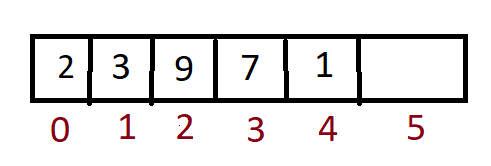Now if we want to insert element 5 at index 3, we need to shift the elements at index 3,4 toward the right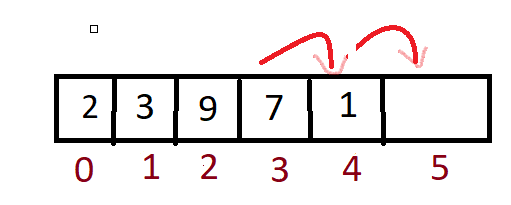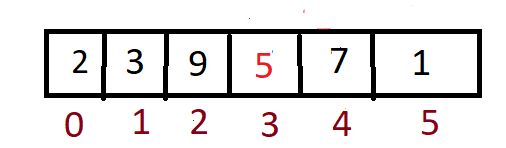Now 5 is inserted, in this way we can insert elements. But what if there was no space to insert a new element? In this case, we will create a new array of size previous array length +1 and will copy all the elements to then we will perform the above-discussed process.

Code:

## C++ Code

``````#include<bits/stdc++.h>
using namespace std;

int main() {
int n =5;
int element=10,index =3;

//Creating array with 1 extra cell so that we can add new element
int arr[n+1] ;

//adding elements to the array
for (int i=0;i<n;i++){
arr[i] = i+2;
}

//moving elements one cell forward till index, starting from last
for (int i=n;i>index;i--){
arr[i] = arr[i-1];
}

//updating element at index(3) to element(10)
arr = element;

for (int i=0;i<n;i++){
cout<<arr[i]<<" ";
}

}``````

Time complexity for inserting elements will be 0(n) because we have to shift all the elements towards the right.

Space complexity: O(n) if a temporary array is created when there is no space in the original array.

## Java Code

``````public class ArrayOperations {
public static void main(String[] args) {
int n =5;
int element=10,index =3;

//Creating array with 1 extra cell so that we can add new element
int arr[] = new int[n+1];

//adding elements to the array
for (int i=0;i<n;i++){
arr[i] = i+2;
}

//moving elements one cell forward till index, starting from last
for (int i=n;i>index;i--){
arr[i] = arr[i-1];
}

//updating element at index(3) to element(10)
arr = element;

for (int i=0;i<n;i++){
System.out.print(arr[i] + " ");
}

}
}
``````

Time complexity for inserting elements will be 0(n) because we have to shift all the elements towards the right.

Space complexity: O(n) if a temporary array is created when there is no space in the original array.

### Replacement/Updation:

Changing/Updating the value at the specified index. This can be easily done by accessing the element at the specified index and assigning a new value to it.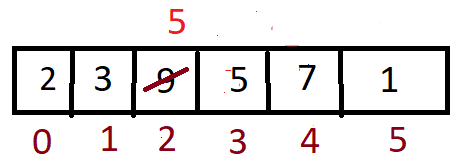In the above array if 9 has to be updated with 5 then we will access 9 using its index

Like array and assign new value i.e 5 to it.

Array = 5;

Code:

## C++ Code

``````#include<bits/stdc++.h>
using namespace std;

int main() {

//updating
int arr[] = {3,2,45,32,12,8};

//updating element 45 which is at index 2 to 100
arr =100;

for (int i=0;i<6;i++){
cout<<arr[i]<<" ";
}

}
``````

## Java Code

``````public class ArrayOperations {
public static void main(String[] args) {

//updating
int arr[] = {3,2,45,32,12,8};

//updating element 45 which is at index 2 to 100
arr =100;

for (int i=0;i<arr.length;i++){
System.out.print(arr[i] + " ");
}

}
}
``````

### Deletion:

Element at any index in the array can be removed/deleted by updating its value by the next element of that index. All the elements have to shift toward the left when an element is deleted in order to fill that gap.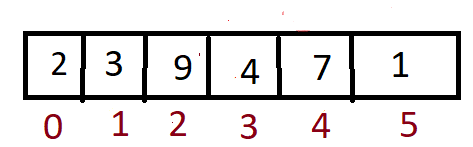Deleting 4 from the above array.

4 is updated with 7 and 7 is updated with 1, this is how deletion works.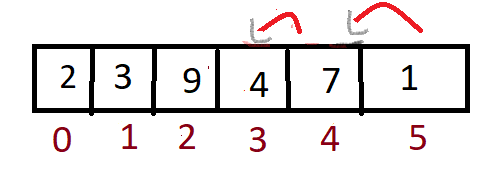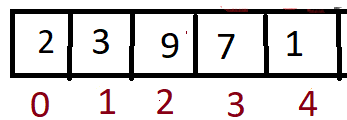Code:

## C++ Code

``````#include<bits/stdc++.h>
using namespace std;
int main()
{
//deleting
int arr[] = {2,3,9,4,7,1};
int n = 6;

//index of element to be deleted
int ind = 3;

// reduce size of array by 1 and move elements one cell
n=n-1;
for (int i=ind;i<n;i++){
arr[i] = arr[i+1];
}

//modified array
for (int i=0;i<n;i++){
cout<<arr[i]<<" ";
}
}

``````

Time complexity: 0(n) – for shifting all the elements to the left by one element.

Space Complexity: O(1)

## Java Code

``````public class ArrayOperations {
public static void main(String[] args) {
//deleting
int arr[] = {2,3,9,4,7,1};
int n = arr.length;

//index of element to be deleted
int ind = 3;

// reduce size of array by 1 and move elements one cell
n=n-1;
for (int i=ind;i<n;i++){
arr[i] = arr[i+1];
}

//modified array
for (int i=0;i<n;i++){
System.out.print(arr[i]+" ");
}
}
}
``````

Time complexity: 0(n) – for shifting all the elements to the left by one element.

Space Complexity: O(1)

### Traversal:

Traversal is to visit all the elements in the array. It is usually used to print all elements, to search,…etc. Traversal is performed using loops.

Code:

## C++ Code

``````#include<bits/stdc++.h>
using namespace std;

int main()
{
//traversing
//visit every element and print
int arr[] = {2,3,9,4,7,1};
int n = 6;

for (int i=0;i<n;i++){
cout<<arr[i]<<" ";
}
}``````

Time Complexity: O(n)

Space Complexity: O(1)

## Java Code

``````public class ArrayOperations {
public static void main(String[] args) {
//traversing
//visit every element and print
int arr[] = {2,3,9,4,7,1};
int n = arr.length;

for (int i=0;i<n;i++){
System.out.print(arr[i]+" ");
}
}
}
``````

Time Complexity: O(n)

Space Complexity: O(1)

### Searching:

Searching can be performed on arrays to search for an element. Mainly two techniques are used to search for an element: Linear search ( Here traversal helps), And Binary search.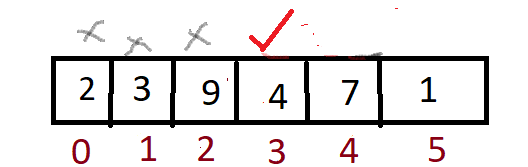Searching using traversal is performed as shown in the above picture. We are searching for 4 so it traverses up to 4 by checking every element. Real-life example: When you login into your bank application may be YONOSBI, it will ask for your credentials, once you enter your mobile number it will show that “Searching for your credentials’ ‘, in this case searching helps a lot.

Code:

## C++ Code

``````#include<bits/stdc++.h>
using namespace std;

int main()
{
//searching
int arr[] = {2,3,9,4,7,1};
int n = 6;
//search for 7
int key =7;
for (int i=0;i<n;i++){
if (arr[i]==key) {
cout<<arr[i]<<" ";
}
}
}

``````

Time Complexity: O(n)

Space Complexity: O(1)

## Java Code

``````public class ArrayOperations {
public static void main(String[] args) {
//searching
int arr[] = {2,3,9,4,7,1};
int n = arr.length;
//search for 7
int key =7;
for (int i=0;i<n;i++){
if (arr[i]==key) {
System.out.print(arr[i] + " ");
}
}
}
}
``````

Time Complexity: O(n)

Space Complexity: O(1)

Note: Searching can also be done using binary search, refer to the article this for that.

## Sorting:

Sorting is performed to sort the data. There are multiple sorting techniques like

Bubble sort, insertion sort, selection sort, merge sort, quick sort……

Real-life example: If you observe your phone contacts application all the contacts are sorted so Sorting techniques will be used here.

Code:

## C++ Code

``````#include<bits/stdc++.h>
using namespace std;

int main()
{
//sorting
int arr[] = {2,3,9,4,7,1};
int n = 6;

//Inbuilt function for sorting
sort(arr,arr+n);

for (int i=0;i<n;i++){
cout<<arr[i]<<" ";
}
}

``````

Time Complexity: O(nlogn)

Space Complexity: O(1)

## Java Code

``````import java.util.*;
public class ArrayOperations {
public static void main(String[] args) {
//sorting
int arr[] = {2,3,9,4,7,1};
int n = arr.length;

//Inbuilt function for sorting
Arrays.sort(arr);

for (int i=0;i<n;i++){
System.out.print(arr[i] + " ");
}
}
}
``````

Time Complexity: O(nlogn)

Space Complexity: O(1)

Special thanks to Sai bargav Nellepalli for contributing to this article on takeUforward. If you also wish to share your knowledge with the takeUforward fam, please check out this article